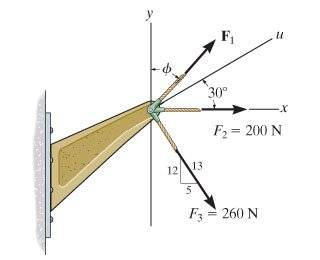# STATICS problem: Minimum Resultant Force

Problem Statement: If the resultant force acting on the bracket is required to be a minimum, determine the magnitude of F1 and the resultant force. Set ∅=30 deg.I have found the components of each force and set them up into a resultant vector, with F1 solved symbolically into its components. I'm not sure how to find the magnitude of F1 so that the resultant is a minimum.

Also, what is the significance of the 'u' axis?

I understand I have to take the derivative of the resultant but I'm not sure how its done. Any help is appreciated, Thanks!

## Answers and Replies

RUber
Homework Helper
You should have something that looks like ##R=(A+x)\hat x + (B + y)\hat y## where x and y are the components of F1. Minimize the magnitude of R.
Using the angles, you should be able to write x and y in terms of magnitude times either sine or cosine to get them to one variable.
Then you can use the standard derivative with respect to magnitude variable to find the minimum.